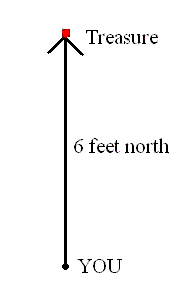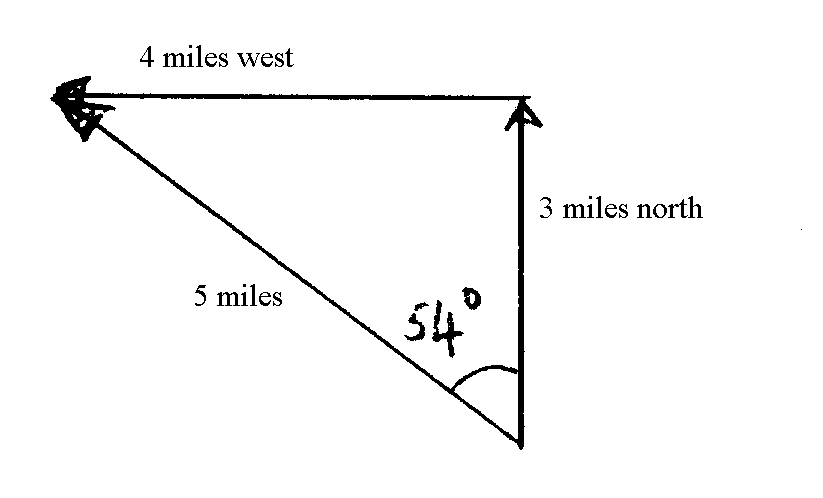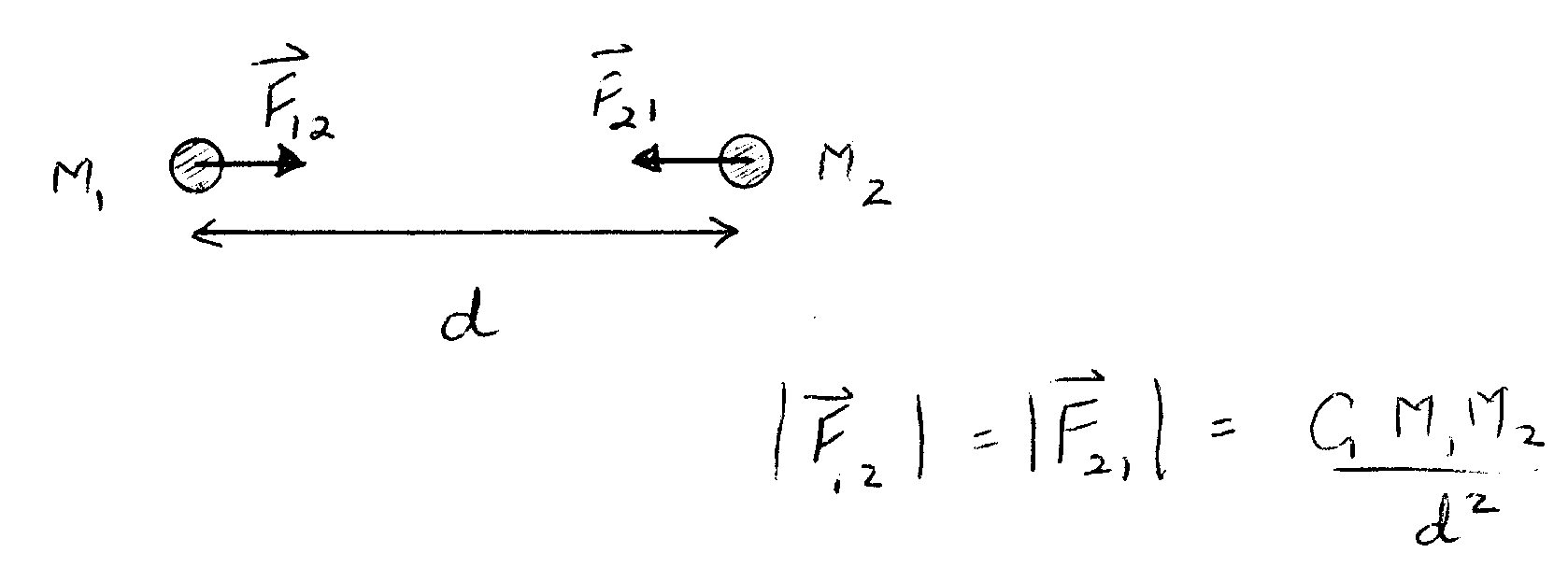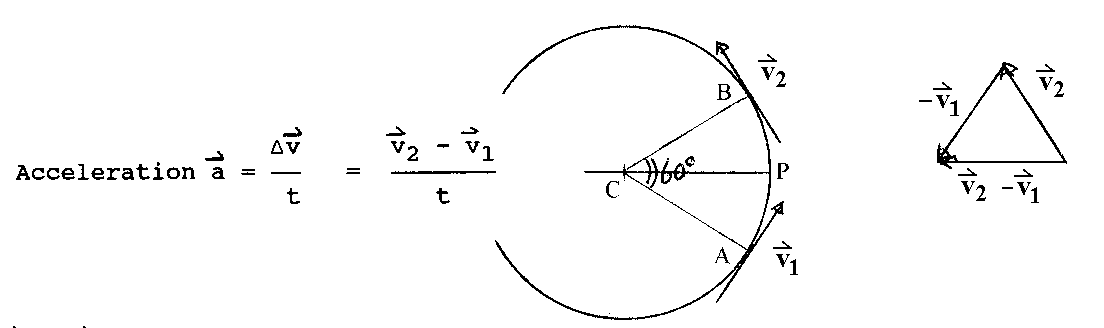# VECTORS

Consider these two statements.
1. The buried treasure is six feet away.
2. The buried treasure is six feet away to the north.
The first tells you something about where the treasure is, but it would take you quite a while to find it. The second statement tells you exactly where to dig!The position of the treasure is described by its position vector. The vector, represented by an arrow, tells you both the distance and the direction of the treasure from you. Many physical quantities have both size and direction, and so must be described by vectors. Examples are velocity (whose size is just speed), acceleration (rate of change of velocity) and magnetic field (as illustrated by a compass needle).

The statement " I walked 3 miles north and then 4 miles west" decscribes two vectors and the process of adding them. Where did you end up? Draw a picture:By Pythagoras theorem, the length of the vector sum (the third side of the triangle) is

square root(9+16) miles = square root(25) miles = 5 miles.
The direction is found by measuring off the triangle or by trigonometry. The answer is 53° 8' West of North.

To add any two vectors we place the tail of the second on the head of the first and then draw a new vector from the tail of the first to the head of the second, as in this diagram.

# NEWTON'S LAWS

1. A particle will remain at rest, or continue in uniform motion in a straight line, unless acted upon by a force.  (In common parlance, a force is a push or a pull.)
2. The acceleration of a body is proportional to the force exerted on it:
3. F = ma,
where m is the mass of the body.
4. Because a force results from the interaction of two objects, forces come in pairs. (For every action there is an equal and opposite reaction.) The forces in the pair are equal in magnitude, opposite in direction, and act on different objects, the two objects that interact.

# NEWTON'S LAW OF GRAVITYThe gravitational force between two bodies is proportional to the mass of each body and inversely proportional to the square of the distance between their centers. The constant of proportionality G is the universal gravitational constant: G = 6.7x10-11 m3/kg.s2.

## WHAT CAUSES CIRCULAR MOTION?

Newton (following ideas of Galileo and Descartes) reasoned that a body in motion will continue to move at constant speed in a straight line unless acted upon by a force. Since circular motion is clearly not in a straight line, we need a force. If the circular motion occurs at constant speed, the force acts only to change the direction of the velocity vector, and not its length. Such a force acts at right angles to the velocity at all times, that is, towards the center of the circle.

#### Examples:

A ball whirled around on the end of a string
The force is provided by the string.
The space shuttle in Earth orbit
The force is provided by the Earth's gravity.
How much acceleration do we need? We can estimate the answer using a diagram. Consider the change in velocity when a particle moves around 1/6 of a circle, i.e. through 60°.`                  delta v      v2 - v1 Acceleration a = -------   =  -------                     t            t`
v2 - v1 points towards the center from P, where P is halfway between A and B. From the drawing, the magnitude of delta v = v = 2(pi)r/T. The time to achieve this change in velocity is 1/6 of the period, ie T/6. Thus the average acceleration during this time has magnitude:
`                 2(pi)  6     12(pi)             a = -----r -  =  ------r                   T    T       T2`
The exact answer, obtained using calculus, is (2pi)2/T2 times r. Our approximate answer is correct to within 5%. Thus the force needed to make the particle move in a circle is mr(2pi/T)2.

## USING NEWTON'S LAWS TO UNDERSTAND KEPLER'S LAWS.

To make life easier, let's assume the planetary orbits are exactly circular instead of almost circular. (It makes the math easier, but doesn't change the result.) We know that the force required for circular motion is mr(2pi/T)2. If gravity provides this force, then:
`    Gmomp       (2pi)2F = ----- = mpr ------      r2          T2`
where mo = mass of sun, mp = mass of the planet, T = period, and r = radius of planet's orbit.
So: T2 = r3 (2pi)2/Gmo, which is just Kepler's third law.